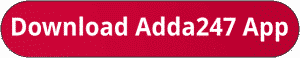Latest Banking jobs   »   Bank Foundation quant

# Quantitative Aptitude Quiz For Bank Foundation 2023-07th October

Directions (1-5): In each of these questions, two equations (I) and (II) are given. You have to solve both the equations and give answer
(a) if x>y
(b) if x≥y
(c) if x<y
(d) if x ≤y
(e) if x = y or no relation can be established between x and y.Directions (6-10): In each of these questions, two equation (I) and (II) are given. You have to solve both the equations and give answer
(a) If x>y
(b) If x≥y
(c) If x<y
(d) If x≤y
(e) If x = y or no relation between x and y.Solutions. .               .                                      .       .

.                                                                                                            .                                                                .                                                      .

## FAQs

### What is the selection process of the Bank Clerk?

The selection process of the Bank Clerk is Prelims & Mains.

#### Congratulations!Union Budget 2023-24: Free PDF